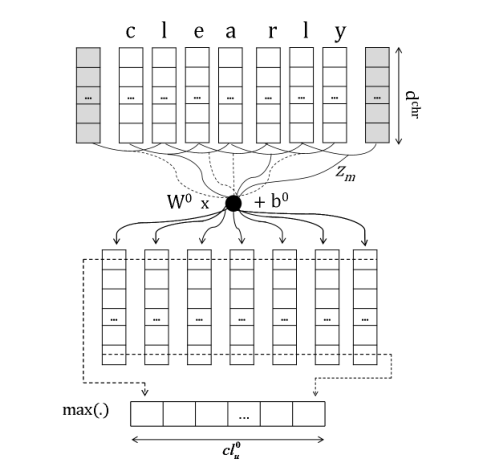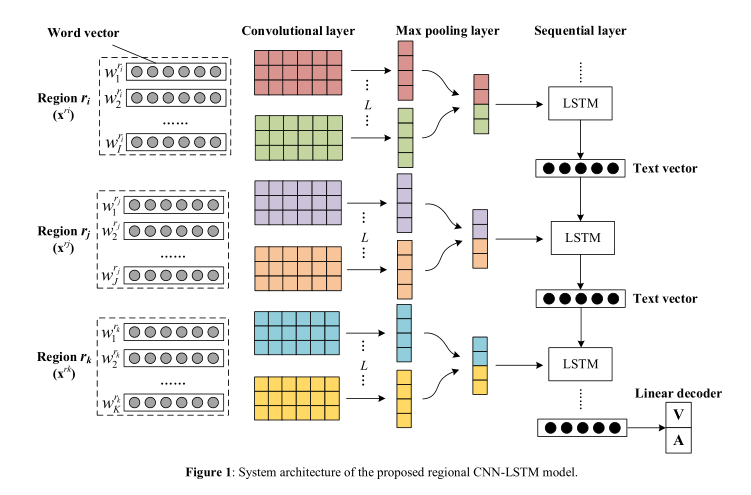# 两个基于神经网络的情感分析模型

## 前言

​情感分析，也称倾向性分析，即对一个主观的文本分析判断说话者的情感色彩或者褒贬态度。传统的方法一般有基于情感词典（构建情感词典后统计文本极性词进行判断）和基于机器学习（使用词袋模型对词语向量化后利用机器学习方法训练分类器），但结果往往差强人意。本周阅读的两篇论文都是基于神经网络对文本进行情感分析，一篇是针对句子级别的文本，另一篇是针对篇章级别的文本。下面进行简单介绍。

### Deep Convolutional Neural Networks for Sentiment Analysis of Short Texts

​本文的主要结构是输入一个句子文本，经过两层CNN转化成一个sentence-level的向量，然后将这个向量输入到一个3层的神经网络中，经过训练得到正确的分类。

#### 1 Word-Level and Character-Level Embeddings

​文中提出一种组合的Word embedings的形式，即把每个词通过word2vec训练得到一个word-level的向量 rwrd$r^{wrd}$，然后把组成每个词的字母字符也训练得到一个character-level的向量rwch$r^{wch}$，这样有利于同时捕捉到语义信息和词形态信息，（比如一些程度副词: terribly，badly ）组合得到一个新的词向量 un=[rwrd,rwch]$u_n = [r^{wrd}, r^{wch}]$

rwrd$r^{wrd}$利用word2vec训练得到的向量表示，rwch$r^{wch}$需要通过一层CNN进行训练得到。思路如下：

​假设词w由M个字母组成，每个字母通过一个character embedding matrix转化成一个向量rchr$r^{chr}$ ，即 rchr=Wchrvc$r^{chr} = W^{chr} v^c$，其中vc$v^c$是one-hot向量，这里类似于word2vec的做法。依次处理后词w就能表示成一个dchrM$d^{chr}*M$ 的向量矩阵了。如下图所示。

​然后使用一个卷积长度为kchr$k^{chr}$ 的过滤器对上述向量进行卷积，再使用一个最大池化层进行池化后得到一个长度为cl0u$cl_u^0$ 的向量，也即rwch$r^{wch}$

​注意到这里卷积的做法和传统卷积不太一样，作者是把相邻几个向量进行拼接之后，通过一个线性计算转化成一个固定维度的向量，感觉是为了统一不同长度词的维度。训练句子级的向量表示也是如此。#### 2 Sentence-Level Embeddings and Scoring

​上述过程后得到句子中每个词的的向量表示[u1,u2,...,uN]$[u_1,u_2,...,u_N]$ ，接下来就是把这N个向量转化成一个句子级别的向量rsent$r^{sent}$ ，具体做法和上述训练rwch$r^{wch}$ 一样。这里不作赘述。得到向量rsent$r^{sent}$后，将向量输入一个三层的神经网络模型中进行训练得到结果。

s=W2h(W1rsent+b1)+b2$s = W_2h(W_1r^{sent} + b_1) + b_2$

#### 3 Results

​模型在两个数据集上进行验证，分别是SSTb和STS数据集。实验结果表示在SSTb数据集上，模型效果优于其他RNN和ML模型，但character-level并不能帮助提升效果。在STS上取得了最好的预测准确率。文章最后还举例说明训练得到的向量能有效识别否定词。

### Dimensional Sentiment Analysis Using a Regional CNN-LSTM Model

Valence表示兴奋或平静的程度, Arousal表示与正面或者负面的程度)

​本文的主要思路是把一个文本按照句子划分成若干个区域，对于每个区域进行卷积池化后得到向量，再把每个区域按照时序关系输入到LSTM中，由LSTM进行encode，最后通过一个linear decoder 进行decode得到预测值。#### 1 Convolutional Layer and Max-pooling Layer

​把文本按照句子划分成K个区域，每个区域里包含句子的词汇，对每个区域进行特征提取并赋予权重，有些区域不含感情色彩则权重会变得很小。每个词汇利用word2vec训练得到的词向量矩阵进行转化为一个向量，即区域i$i$ 可以表示为ri=[wri1,wri2,...,wriI]$r_i = [w_1^{r_i},w_2^{r_i},...,w_I^{r_i}]$ 这样一个矩阵，然后再利用L个卷积过滤器对其进行卷积，卷积窗口长度为ω$ω$ ，即过滤器l$l$ 进行一次窗口卷积后将得到一个映射值yn$y_n$ ，最后得到向量yl=yl1,yl2,...,ylNω+1$y^l = y_1^l,y_2^l,...,y_{N-ω+1}^l$

yln=f(Wlxn:n+ω1+bl)$y_n^l = f(W^l ○ x_{n:n+ω-1} + b^l)$

​向量yl$y^l$ 再进行最大池化层进行池化，得到新的向量。

#### 2 LSTM Encoder and Linear Decoder

​ 由于篇章级文本存在时序关系和长距离依赖关系，所以可以利用LSTM把上述得到的区域向量当作一个序列进行编码，最终得到的hidden state 可以当作是文本的表示形式 xt$x_t$ 。因为VA空间两个维度上的值都是连续值，这里需要一个回归模型进行回归，所以使用一个线生的解码器进行回归，最终得到预测值。即：

y=Wdxt+bd$y = W_d x_t + b_d$

#### 3 Results

​ 模型在英文数据集SST和中文数据集CVAT上进行验证，实验表明在两个数据集上，模型效果都比传统方法和不加区域化的CNN和LSTM等方法都更好。

## 总结

​两篇论文都使用了神经网络对不同级别的文本进行情感分析。论文一主要提出了一个基于character的sub embedding，虽然提升效果不是很明显，但提取词形特征这个想法还是值得思考的。卷积层在调整向量固定长度时似乎有点矛盾，这点还需要讨论。论文二主要提出分两步（CNN-LSTM）对文本进行分析，前面CNN与论文一相似，主要在于后面一层，采用的是把所有句子当作一个序列输入到LSTM学习，保证了前后时序关系，我认为这点对篇章级的文本分析比较重要。

## 参考资料

• Santos C N D, Gattit M. Deep Convolutional Neural Networks for Sentiment Analysis of Short Texts[C]// International Conference on Computational Linguistics. 2014.

• Wang J, Yu L C, Lai K R, et al. Dimensional Sentiment Analysis Using a Regional CNN-LSTM Model[C]// Meeting of the Association for Computational Linguistics. 2016:225-230.